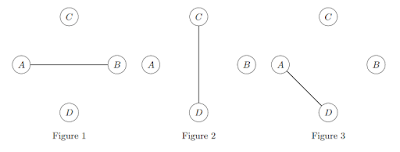## Tuesday, August 16, 2016

### Crypto 2016: Network Oblivious Transfer

On the first day of CRYPTO 2016, Adam Sealfon presented his work with Ranjit Kumaresan and Srinivasan Raghurama on Network Oblivious Transfer. Oblivious transfer (OT) is a two party protocol in which party $A$ inputs two strings and party $B$ a bit $b$: $B$ receives exactly one of the strings according to his bit and finds out nothing about the other string, while $A$ does not find out which of the two strings $B$ chose. If two parties are able to engage in an OT protocol, we say that there is an OT channel between them. OT channels are a good thing to study because they are:
• Useful: OT has been called MPC (multi-party computation) complete, and the Atom of MPC, since many MPC protocols can be realised using OT;
• Achievable: e.g. trapdoor permutations can be used to realise them.
Suppose we have a network in which all parties have secure connections to all other parties, and some of the parties also have OT channels between them. What can we say about the ability of the network to allow computation of OT-based MPC? In 2007, Harnik et al. asked How Many Oblivious Transfers are Needed for Secure Multiparty Computation? and give a lower bound on the number of OT channels a network must have. The paper presented gave an upper bound which matches the lower bound of the aforementioned paper, and hence allows a complete characterisation of the networks in which OT channels can be established to enable secure MPC.

For some intuition as to what this all means, consider the following three graphs. Nodes represent parties in the network, and edges represent OT channels. All parties are assumed to have secure connections to all other parties and we want to have an OT channel between $A$ and $B$.In Figure 1, $A$ and $B$ have an OT channel between them, so we're done. In Figure 2, it turns out that the connections in place already suffice to provide $A$ and $B$ with an OT channel. However, in Figure 3, we cannot form an OT channel between $A$ and $B$.

The reason some graphs admit OT channels between certain parties and some do not concerns a property known as splittability. A graph $G$ is called $k$-unsplittable (for $k<n/2$) if for any two disjoint sets of $k$ vertices, there is an edge from a vertex in one set to a vertex in the other; $G$ is called $k$-splittable if this does not hold. The main theorem of the paper states that, assuming a semi-honest adaptive adversary controlling no more than $t$ parties, two parties, $A$ and $B$, in the network can establish an OT channel if and only if
1. $t<n/2$, or
2. $t \ge n/2$ and the graph is $(n-t)$-splittable.
Adding the edge $(A,B)$ to Figures 2 and 3 shows this at least looks like it says the right thing, since doing so in Figure 3 shows every 2-split of the graph has an edge between the two partitions.

In proving this theorem, the paper provides a good description of the limits of OT-based MPC.